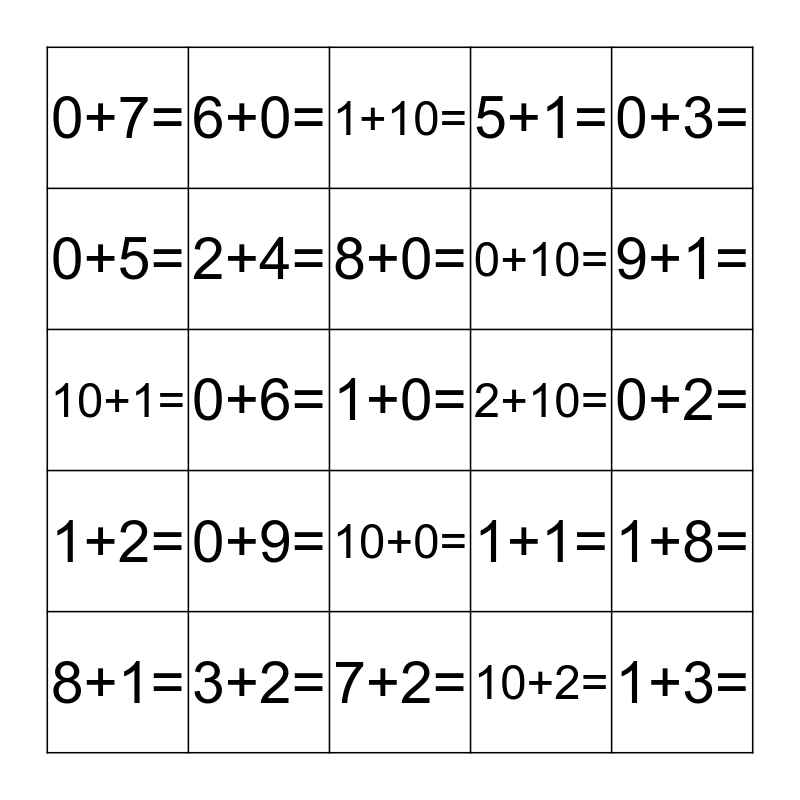# Name: __________________________This bingo card has 68 words: 0+0=, 1+0=, 2+0=, 3+0=, 4+0=, 5+0=, 6+0=, 7+0=, 8+0=, 9+0=, 10+0=, 0+1=, 0+2=, 0+3=, 0+4=, 0+5=, 0+6=, 0+7=, 0+8=, 0+9=, 0+10=, 1+1=, 1+2=, 1+3=, 1+4=, 1+5=, 1+6=, 1+7=, 1+8=, 1+9=, 1+10=, 2+1=, 3+1=, 4+1=, 5+1=, 6+1=, 7+1=, 8+1=, 9+1=, 10+1=, 2+2=, 2+3=, 2+4=, 2+5=, 2+6=, 2+7=, 2+8=, 2+9=, 2+10=, 3+2=, 4+2=, 5+2=, 6+2=, 7+2=, 8+2=, 9+2=, 10+2=, 3+3=, 3+7=, 7+3=, 4+4=, 4+6=, 6+4=, 5+5=, 6+6=, 7+7=, 8+8= and 9+9=.

## Play Online